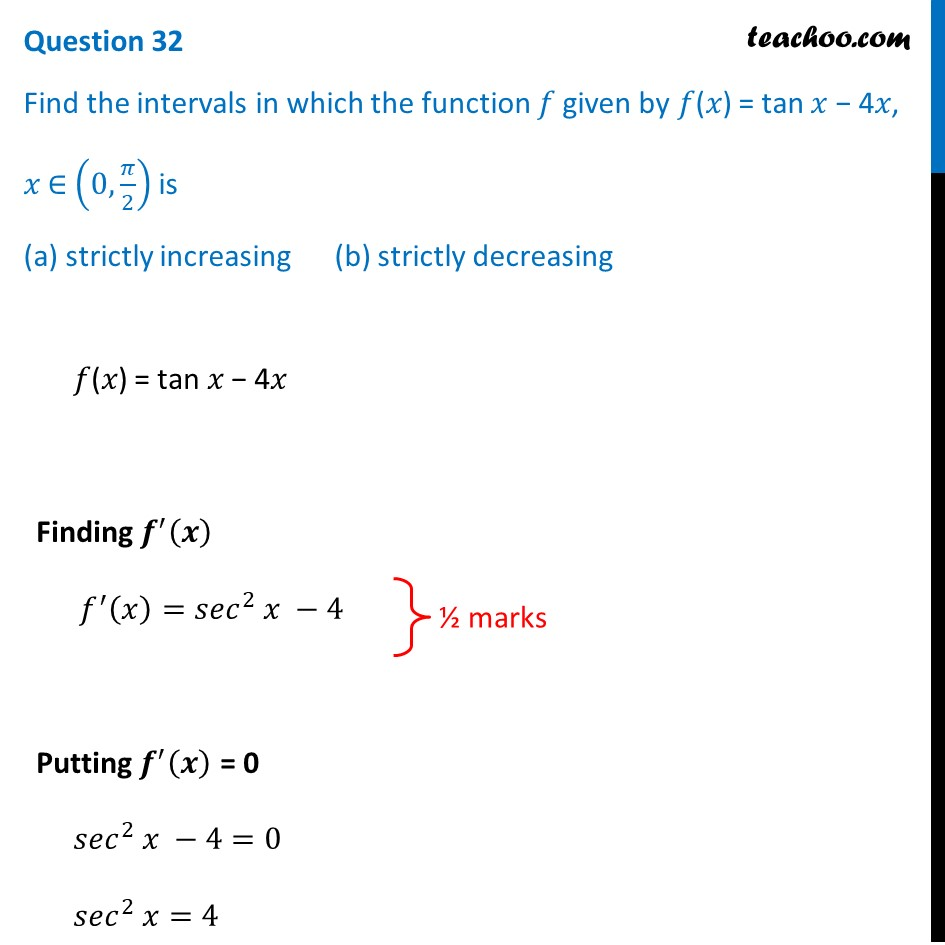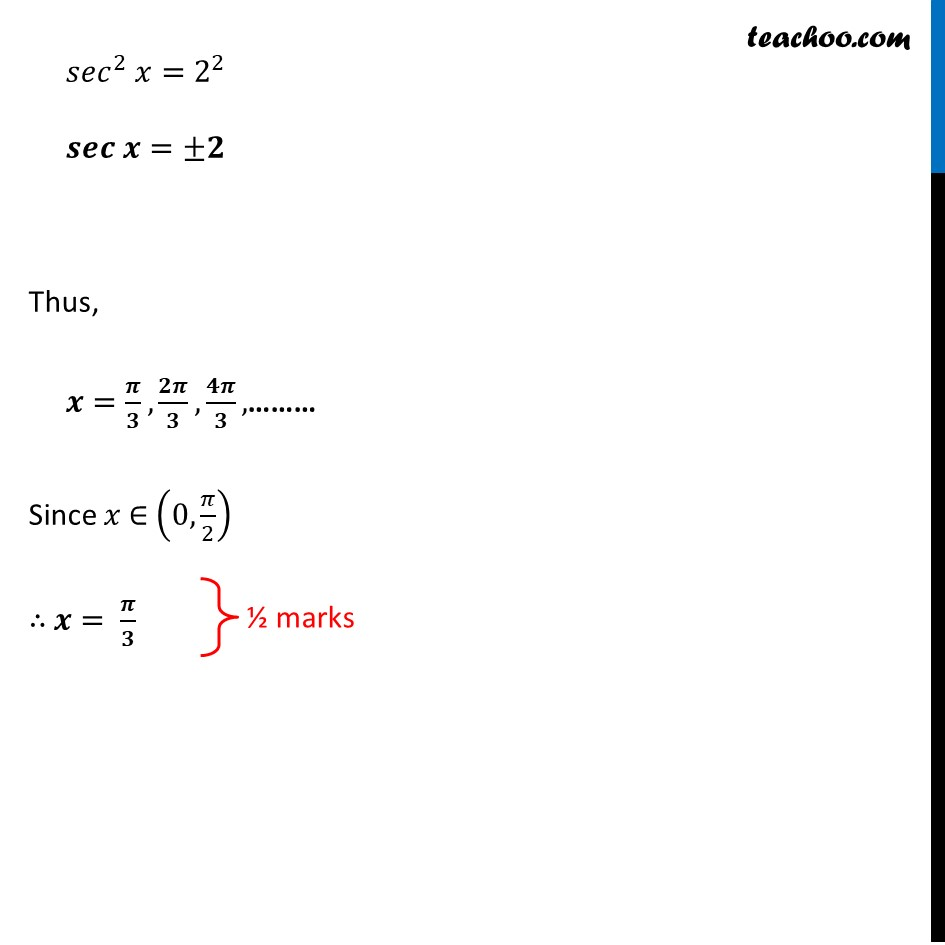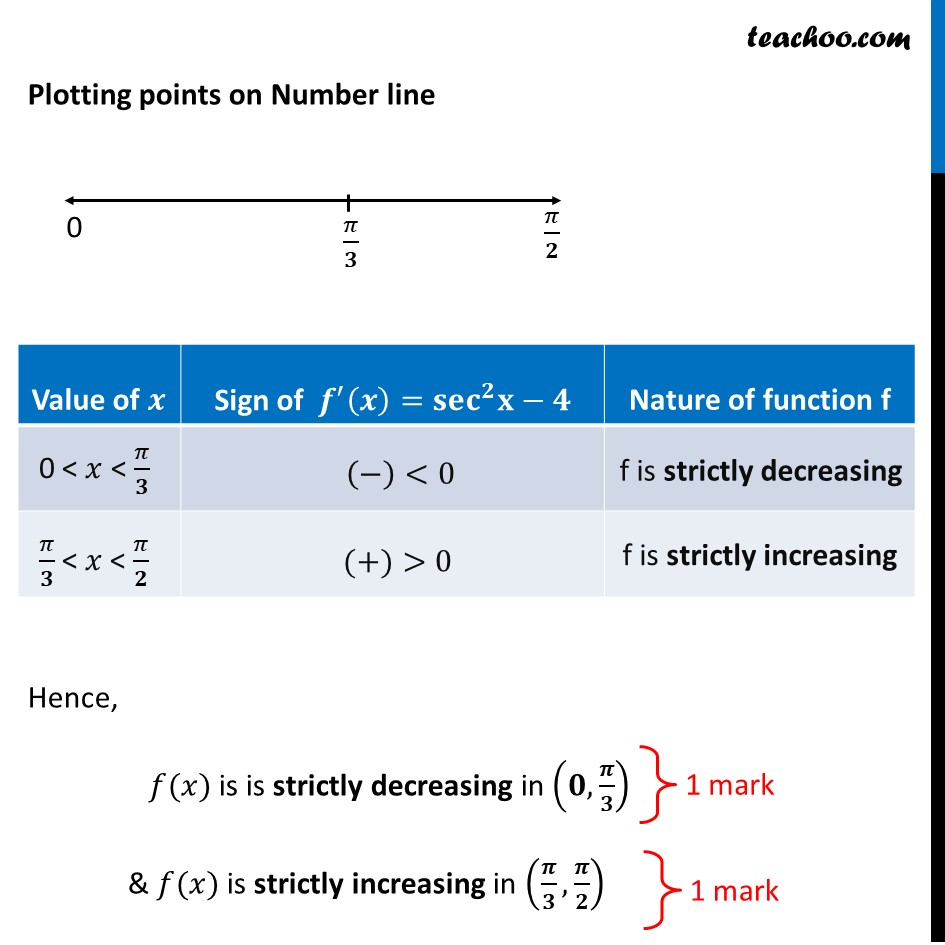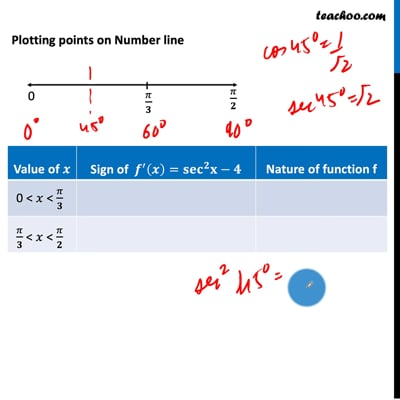CBSE Class 12 Sample Paper for 2021 Boards

Class 12
Solutions of Sample Papers and Past Year Papers - for Class 12 Boards

## Find the intervals in which the function 𝑓 given by 𝑓(𝑥) = tan 𝑥 − 4𝑥, 𝑥 ∈ (0,π/2) is (a) strictly increasing      (b) strictly decreasingThis video is only available for Teachoo black users

Introducing your new favourite teacher - Teachoo Black, at only ₹83 per month

### Transcript

Question 32 Find the intervals in which the function 𝑓 given by 𝑓(𝑥) = tan 𝑥 − 4𝑥, 𝑥 ∈ (0,𝜋/2) is (a) strictly increasing (b) strictly decreasing 𝑓(𝑥) = tan 𝑥 − 4𝑥 Finding 𝒇^′ (𝒙) 𝑓^′ (𝑥)=〖𝑠𝑒𝑐〗^2 𝑥 −4 Putting 𝒇^′ (𝒙) = 0 〖𝑠𝑒𝑐〗^2 𝑥 −4=0 〖𝑠𝑒𝑐〗^2 𝑥=4 〖𝑠𝑒𝑐〗^2 𝑥=2^2 𝒔𝒆𝒄 𝒙=±𝟐 Thus, 𝒙=𝝅/𝟑,𝟐𝝅/𝟑,𝟒𝝅/𝟑,……… Since 𝑥 ∈ (0,𝜋/2) ∴ 𝒙= 𝝅/𝟑 Plotting points on Number line Hence, 𝑓(𝑥) is is strictly decreasing in (𝟎,𝝅/𝟑) & 𝑓(𝑥) is strictly increasing in (𝝅/𝟑,𝝅/𝟐)